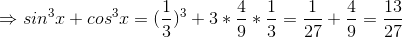# High School Math : Trigonometric Equations

## Example Questions

### Example Question #1 : Solving Trigonometric Equations

If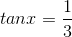, find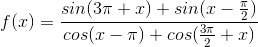.

1

-2

-1

0

2

2

Explanation:

First we need to simplify each term: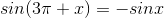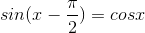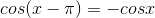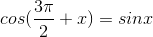So we will have: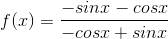Since we have the value of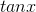, we can divide the numerator and denominator by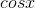.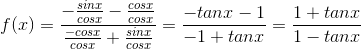We can then substitute the value ofin the obtained function.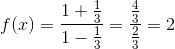### Example Question #2 : Trigonometric Equations

A right triangle has an angle of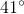. If the side opposite it has a length of, what is the hypotenuse?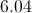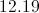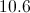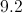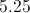Explanation:

For this problem we will use the sine function as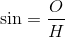.

Plug in our given information: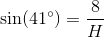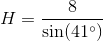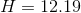### Example Question #3 : Trigonometric Equations

A right triangle has an angle of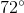. If the side adjacent to it has a length of, what is the hypotenuse?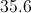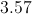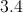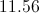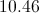Explanation:

For this problem we will use the cosine function as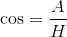.

Plug in our given information: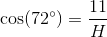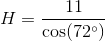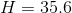### Example Question #4 : Trigonometric Equations

In a right triangle, if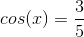and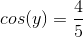then what does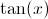equal?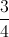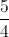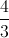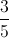Explanation:

One can draw a right triangle with acute angles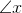and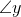. The side adjacent tois 4, and the side adjacent tois 3.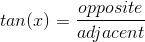### Example Question #5 : Trigonometric Equations

If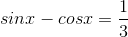, find the value of the function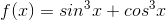.

1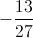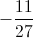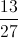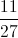Explanation:

We can write: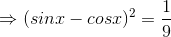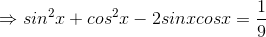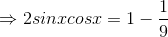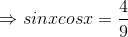We can also write: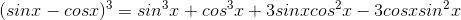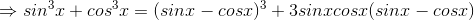Now we can substitute the values of (sinx - cosx) and (sinx * cosx) in the obtained function: